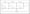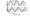# GTU Question papers 2011 Elements of Electrical Engineering

GUJARAT TECHNOLOGICAL UNIVERSITY B.E. Sem-I/II

Examination June-July 2011

Subject code: 110005

Subject Name:  Element of Electrical Engineering

Instructions:

1. Attempt all questions.
2. Make suitable assumptions wherever necessary.
3. Figures to the right indicate full marks.

Q.1 (a) Explain the factors affecting the resistance of a conducting material.

(b)        An alternating voltage of v = 100sin(376.8 t) is applied to a coil having resistance 6 Q and inductance of 21.22 mH.

Determine (i) Current through the coil

(ii) Power dissipated in the coil

(c)        The waveform for instantaneous power in ac circuit is resolved into two component, i.e. p(t)=pi(t)+p2(t). Plots of both components are shown in fig no1 . Calculate magnitude of total real power and magnitude of total reactive power (write unit also).

Q.2 (a) Explain charging and discharging of a capacitor, C, through a resistor, R, with neat sketch and derive the equation Vc = V (1- e-t/RC). Assume that the R-C series circuit is connected across a d.c supply of voltage V.

(b) A parallel plate capacitor has plates of area 2 m2 spaced by three slabs of different materials .The relative permittivity are 2,3 and 6 and thickness are 0.4, 0.6 and 1.2 mm respectively. Calculate the equivalent capacitance and electric stress in each material when the applied voltage is 1000 V.

OR

(b) A ring has a mean diameter of 21 cm and a cross sectional area of 10 cm2. The ring is made up of semi circular sections of cast iron and cast steel, with joint having reluctance equal to an air gap of 0.2 mm. Find the amp-turns required to produce a flux of 8 X 10″4 wb. The relative permeability of cast steel and cast iron are 800 and 166 respectively. Neglect fringing and leakage effect

Q.3 (a) State and explain self inductance (L). Derive expression of co-efficient of mutual coupling between two coils having self inductances of L1 and l2.

(b)           Explain magnetic hysteresis

(c)           Prove that the average power consumption in a pure inductive or capacitive circuit is zero.

OR

Q.3 (a) A balanced three phase supply is given to a star connected load. Give proof of two wattmeter method for this system. State demerits of this method.

(b)        Derive the equation of energy stored in a magnetic field.

(c)        An alternating current having an equation I =141.4sin314t.

Find: (i)Frequency (ii)R.M.S. Value (iii)Average Value (iv) An instantaneous value of current when ‘ t’ is 2 ms.

Q.4 (a) State and Explain plate and pipe earthling with neat diagram.                                07

(b) General guideline for wiring of domestic installation with neat sketch 07 for position of equipment.

OR

Q.4 (a) Explain with diagram different types of illumination scheme.                               07

Explain with neat sketch construction and working of lead acid battery                 7

Q.5 (a) Explain with neat sketch general construction of cable.

(b)           With necessary diagram derive the formula for star to delta and delta to star transformation.

(c)           A balanced three phase power supply is connected to a balanced load. Derive the expression of instantaneous power of phase A ,

pa(t). Obtain expression of p(t), where p(t) is summation of instantaneous powers of all three phases. assume that va(t)=Vmsinrot and ia (t)=I msin(rot-0).

OR

Q.5 (a) Determine the current in 17 Q resistor in the network shown in Figure 07Give comparison of series and parallel resonance in R-L-C A.C circuit. Derive expression of quality factor, Q for series R-L-C circuit.